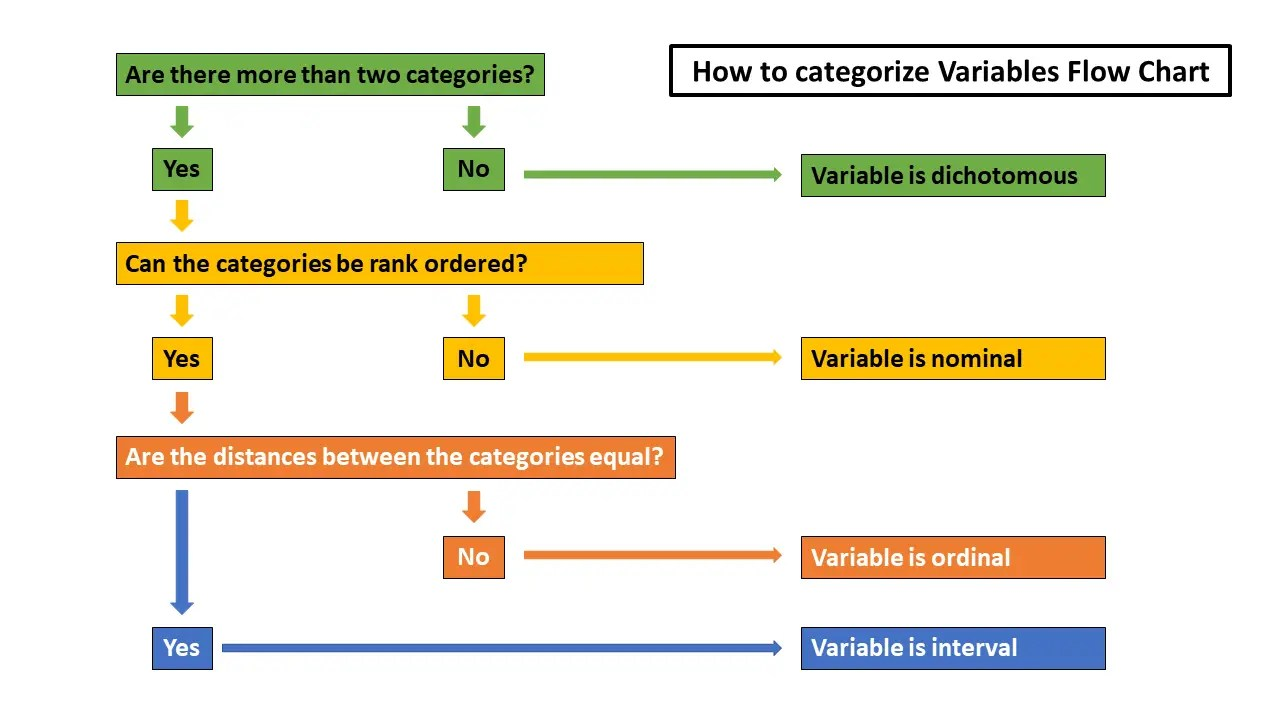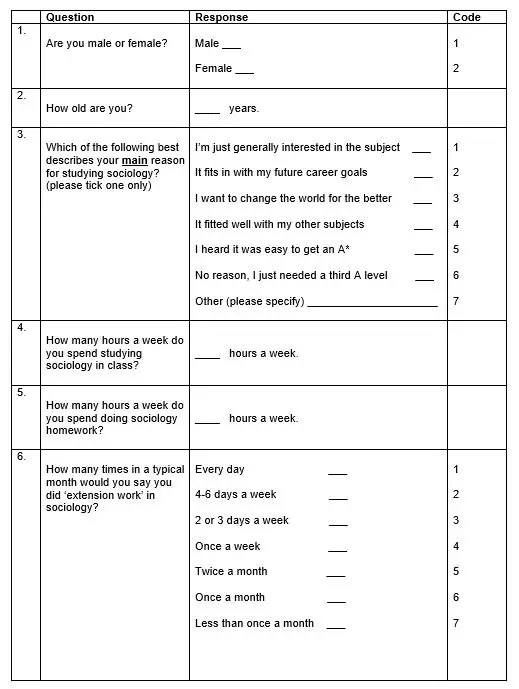## Variables in quantitative reserach

What is the difference between interval/ ratio, ordinal, nominal and categorical variables? This post answers this question!#### Interval/ ratio variables

Where the distances between the categories are identical across the range of categories.

For example, in question 2, the age intervals go up in years, and the distance between the years is same between every interval.

Interval/ ratio variables are regarded as the highest level of measurement because they permit a wider variety of statistical analyses to be conducted.

There is also a difference between interval and ratio variables… the later have a fixed zero point.

#### Ordinal variables

These are variables that can be rank ordered but the distances between the categories are not equal across the range. For example, in question 6, the periods can be ranked, but the distances between the categories are not equal.

NB if you choose to group an interval variable like age in question 2 into groups (e.g. 20 and under, 21-30, 31-40 and so on) you are converting it into an ordinal variable.

#### Nominal or categorical variables

These consist of categories that cannot be rank ordered. For example, in questions 7-9, it is not possible to rank subjective responses of respondents here into an order.

#### Dichotomous variables

These variables contain data that have only two categories – e.g. ‘male’ and ‘female’. Their relationship to the other types of variable is slightly ambiguous. In the case of question one, this dichotomous variable is also a categorical variable. However, some dichotomous variables may be ordinal variables as they could have one distinct interval between responses – e.g. a question might ask ‘have you ever heard of Karl Marx’ – a yes response could be regarded as higher in rank order to a no response.

Multiple-indicator measure such as Likert Scales provide strictly speaking ordinal variables, however, many writers argue they can be treated as though they produce interval/ ratio variables, if they generate large number of categories.

In fact Bryman and Cramer (2011) make a distinction between ‘true’ interval/ ratio variables and those generated by Likert Scales.

### A flow chart to help define variables*A nominal variable – aka categorical variable!

### Questionnaire Example

This section deals with how different types of question in a questionnaire can be designed to yield different types of variable in the responses from respondents.

If you look at the example of a questionnaire below, you will notice that the information you receive varies by questionSome of the questions ask for answers in terms of real numbers, such as question 2 which asks ‘how old are you’ or questions 4 and 5 and 6 which asks students how many hours a day they spend doing sociology class work and homework. These will yield interval variables.

Some of the questions ask for either/ or answers or yes/ no answers and are thus in the form of dichotomies. For example, question 1 asks ‘are you male or female’ and question 10 asks students to respond ‘yes’ or ‘no’ to whether they intend to study sociology at university. These will yield dichotomous variables.

The rest of the questions ask the respondent to select from lists of categories:

The responses to some of these list questions can be rank ordered – for example in question 6, once a day is clearly more than once a month! Responses to these questions will yield ordinal variables.

Some other ‘categorical list’ questions yield responses which cannot be ranked in order – for example it is impossible to say that studying sociology because you find it generally interesting is ranked higher than studying it because it fits in with your career goals.  These will yield categorical variables.

These different types of response correspond to the four main types of variable above.